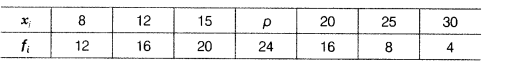# If we divide each frequency of above data by 2, then check whether the value of p

If we divide each frequency of above data by 2, then check whether the value of p will be same as above.The value of p will remain same, on dividing each frequency by 2.